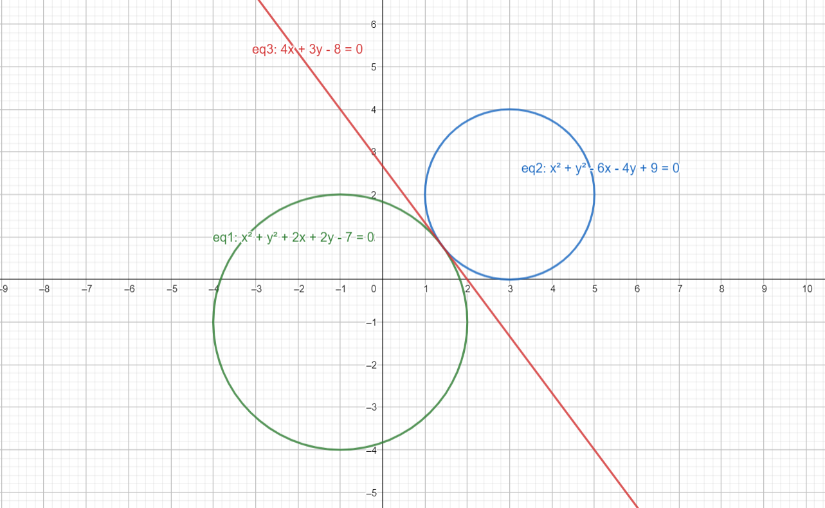Courses
Courses for Kids
Free study material
Free LIVE classes
MoreLIVE
Join Vedantu’s FREE Mastercalss

# Statement 1Given two circles ${{S}_{1}}$=0 and ${{S}_{2}}$=0 having same coefficients in ${{x}^{2}}$and ${{y}^{2}}$ then ${{S}_{1}}-{{S}_{2}}=0$ is the equation of a common tangent of the circles.Statement 2$4x+3y-8=0$ is a common tangent of the circles ${{x}^{2}}+{{y}^{2}}+2x+2y-7=0$ and ${{x}^{2}}+{{y}^{2}}-6x-4y+9=0$(a) Statement -1 is True, Statement 2 is True; Statement 2 is a correct explanation for Statement 1(b) Statement 1 is True, Statement 2 is true; Statement 2 is NOT a correct explanation for Statement 1(c) Statement 1 is True, Statement 2 is False(d) Statement 1 is False, Statement 2 is TrueVerified
363.9k+ views
Hint: The radical axis of two circles that have the same coefficients in ${{x}^{2}}$and ${{y}^{2}}$ is equal to the difference of their two equations. But the radical axis becomes the common tangent as well, only when the circles touch each other at exactly one point. In all other cases, it’ll just be a radical axis and nothing else.

To find common tangents between two circles, find out the number of common tangents they’ll have and then proceed by finding the point of intersection of the common tangents, from where you can assume a general equation and start working.

For Statement 1, it is said that if two circle equations have same coefficient of ${{x}^{2}}$ and ${{y}^{2}}$, it means that their common tangent can be found by finding the difference between the two circle equations.
This is not always true.
${{S}_{1}}-{{S}_{2}}=0$ always gives us the equation of a radical axis of two circles. However, this radical axis is the same as their common tangent if and only if the two circles touch each other at exactly one point, externally, i.e. one circle doesn’t lie inside the other circle.
In all other cases, it is not necessary that the radical axis or the equation of the line obtained from ${{S}_{1}}-{{S}_{2}}=0$ is the common tangent as well.
Hence, Statement 1 is False.
Let’s call the circle as ${{S}_{1}}$: ${{x}^{2}}+{{y}^{2}}+2x+2y-7=0$ and the circle ${{x}^{2}}+{{y}^{2}}-6x-4y+9=0$ as ${{S}_{2}}$.
The general equation of any circle can be written as :
${{x}^{2}}+{{y}^{2}}+2gx+2fy+c=0$where the circle’s centre $C=(-g,-f)$ and its radius $r=\sqrt{{{g}^{2}}+{{f}^{2}}-c}$
Therefore, for circle ${{S}_{1}}$, comparing the circle’s equation with the general form, we get :
\begin{align} & 2gx=2x \\ & \Rightarrow g=1 \\ \end{align}
And, \begin{align} & 2fy=2y \\ & \Rightarrow f=1 \\ \end{align}
Therefore, the centre of circle ${{S}_{1}},{{C}_{1}}=(-g,-f)=(-1,-1)$
and its radius ${{r}_{1}}=\sqrt{{{g}^{2}}+{{f}^{2}}-c}$ = $\sqrt{{{1}^{2}}+{{1}^{2}}+7}=\sqrt{9}=3$

For the circle ${{S}_{2}}$, we again compare its equation with the general form of circles, and see that :
\begin{align} & 2gx=-6x \\ & \Rightarrow g=-3 \\ \end{align}
And, \begin{align} & 2fy=-4y \\ & \Rightarrow f=-2 \\ \end{align}
Therefore, the centre of circle ${{S}_{2}},{{C}_{2}}=(-g,-f)=(3,2)$
And its radius ${{r}_{2}}=\sqrt{{{g}^{2}}+{{f}^{2}}-c}$= $\sqrt{9+4-9}=\sqrt{4}=2$

For two circles to touch each other at exactly one point, it is necessary that the distance between their centres = sum of their radii.
If $({{r}_{1}}+{{r}_{2}})=|{{C}_{1}}-{{C}_{2}}|$, this means that the circles intersect at exactly one point.
$({{r}_{1}}+{{r}_{2}})=5$ and $|{{C}_{1}}-{{C}_{2}}|=\sqrt{{{(-1-3)}^{2}}+{{(-1-2)}^{2}}}=\sqrt{{{4}^{2}}+{{3}^{2}}}=5$
$\Rightarrow ({{r}_{1}}+{{r}_{2}})=|{{C}_{1}}-{{C}_{2}}|$
This means that the circles touch each other at exactly one point, and thus, their transverse common tangent will be the same as their radical axis.
The radical axis of the two circles is found by finding the difference of their equations provided the coefficients of ${{x}^{2}}$ and ${{y}^{2}}$ are equal.
Since the coefficients of ${{x}^{2}}$ and ${{y}^{2}}$are equal to $1$ in both ${{S}_{1}}$ and ${{S}_{2}}$,
Their transverse common tangent $={{S}_{1}}-{{S}_{2}}=0$
Therefore, required equation $={{S}_{1}}-{{S}_{2}}=0$
Substituting for ${{S}_{1}}$ and ${{S}_{2}}$ in the equation, we get :
\begin{align} & {{x}^{2}}+{{y}^{2}}+2x+2y-7-({{x}^{2}}+{{y}^{2}}-6x-4y+9)=0 \\ & \Rightarrow 8x+6y-16=0 \\ \end{align}
Dividing both sides by $2$, we get :
$\Rightarrow 4x+3y-8=0$
And this is the equation of their common tangent.
To ease your understanding here is a diagram of the two circles drawn to scale exactlyIn the diagram, eq3 shows the equation or the common tangent given in the question. Hence, we can see that this line acts as a tangent to both the circles.
Hence, Statement 2 is True.
Hence, answer is (d) Statement 1 is False, Statement 2 is True.

Note: In this question, after proving that the circles touch each other and would have only one tangent, you could also find the common tangent by adopting the general method where we assume the equation of the tangent as $y=mx+c$. However, keep in mind that this tangent will cut both the circles at the same point, so we can make the coordinates of the point satisfy the general equation, and then apply the condition of the perpendicular distance from the centre of the circle being equal to the circle’s radius.
Last updated date: 29th Sep 2023
Total views: 363.9k
Views today: 6.63k